# More Handshake Problems

Last week we looked at problems about counting diagonals in a polygon, and the very similar problem of counting handshakes when everyone in a group shakes with everyone else. In the course of searching for those problems, I also found some very different problems that are also about handshakes. We’ll look at those here, just for fun.

## How many people?

First, let’s look at a question from 1996 that just reverses the problem:

Handshakes at a Party

If there is a party and every person shakes hands with each other
once, and there are 45 handshakes, how many people are there at the
party?

I don't have a clue how to solve it.

Doctor Anthony answered, by first deriving the formula as we’ve seen before:

If there are n people at the party, then each person will shake hands
with n-1 other people.  So with n people each making (n-1) handshakes,
it appears at first sight that there are n(n-1) handshakes.

However, each handshake will have been counted twice, i.e. A->B and
B->A, so we must divide by 2.

Total number of handshakes = n(n-1)/2

But this time, we don’t know n! That’s what we have to find:

Now we are given that there were 45 handshakes in all, so we must solve the equation:

n(n-1)/2 = 45

n(n-1) = 90

n^2 - n - 90 = 0

(n-10)(n+9) = 0      From this n = 10 or -9

Clearly the -9 has no meaning in this question, so we conclude that n = 10.

Number at the party = 10.

To check, each of the 10 people shakes 9 hands, for a total of 90 hands involved in shakes; divide by two for the actual number of shakes, and we have 45.

If there were no positive integer solution, then we would know that the handshakes had been miscounted (or the whole thing was made up with random numbers!). Only certain numbers can occur as the number of handshakes (when everyone shakes with everyone else); we could call these “handshake numbers”. But that would be yet another problem …

## Everyone with a different number

Now we’ll look at some entirely different problems, starting with this from 1999:

Handshake Problem Variant

Taking the handshake question further:

Ten people (five couples) go to a party and start shaking hands. You don't shake your spouse's hand or (of course) your own. One of the men, Jake, shouts, "Stop! How many hands did you shake?" Every person gives a different answer. Jake's wife is the last to answer. How many handshakes does she have? Is it 0?

Dear Stephanie,

Nine possible answers? Yes, people can shake hands with 8 people or fewer, and thus the possible answers are 0, 1, 2, 3, 4, 5, 6, 7 or 8.

We’re interpreting the problem to say that Jake himself was among those who all gave different answers, so that his wife is the tenth; if he didn’t answer his own question, so there were only 9 answers (including his wife), then the problem would be unanswerable. As we’re taking it, the 9 other than his wife (including himself) use up all 9 possible answers, and then his wife gives a tenth answer (which has to match one of the others).

Here are the ten people, with couples joined by blue lines: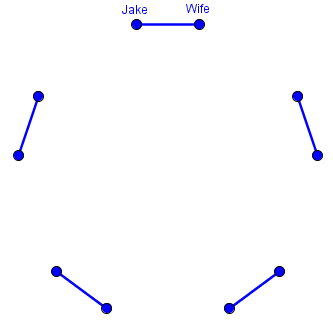The person who answers 8 has shaken hands at least with the persons that have answered 1, 2, ..., 7. That's only 7 persons, so she or he has shaken hands with Jake's wife too. This also means that the person who answers 0 is the only one who has not shaken hands with the person who answered 8, so they must be a couple.

So we have something like this, where red lines represent handshakes known so far: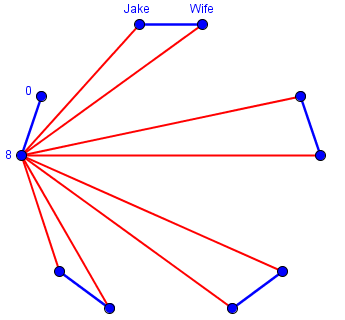Person 8 shook hands with everyone except himself and his wife, which is as many as possible.

We can now leave out this couple. As a thought experiment, we could re-ask the question restricting ourselves to the 8 people left. Originally, the 7 persons in this group answered 1, 2, 3, 4, 5, 6, 7. All these people have shaken hands with the person who answered 8 and none of them has shaken hands with the person who answered 0. So when restricting themselves to the new group, they would have answered: 0, 1, 2, 3, 4, 5 and 6.

We’re starting over with only 8 people, and ignoring the handshake each of them would have had with Person 8: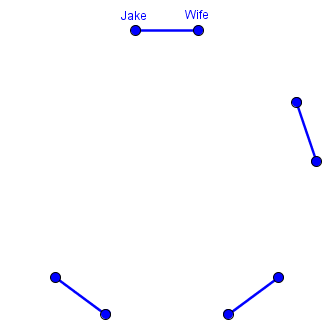We can now repeat our argument:

The person who now answers 6 has shaken hands with Jake's wife. The persons who answer 0 and 6 form a couple. We can leave out this couple.

This couple answered 1 and 7 to the actual question, so we now add these shakes: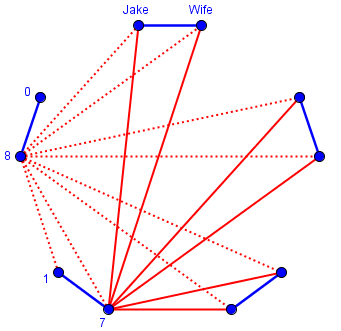Person 7 shook hands with Person 8 and 6 others.

This leaves 6 people. When restricting themselves to the new group, they would have answered: 0, 1, 2, 3 and 4. The person who now answers 4 has shaken hands with Jake's wife. The persons who answer 0 and 4 form a couple. We can leave out this couple.

They answered 2 and 6, when we add back the two handshakes we ignored: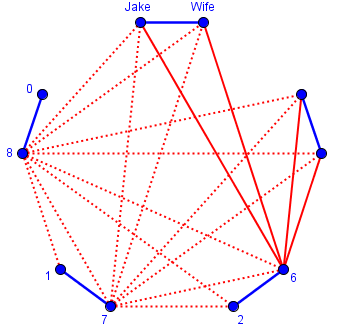This leaves 4 people. When restricting themselves to the new group, they would have answered: 0, 1 and 2. The person who answers 2 has shaken hands with Jake's wife (and with Jake). The persons who answer 0 and 2 form a couple. The other couple is Jake and his wife.

We can conclude that Jake's wife has shaken hands with 4 people.

Here is the final round, adding in the people who shook 3 and 5 hands in all, and counting the hands Jake and his wife shook: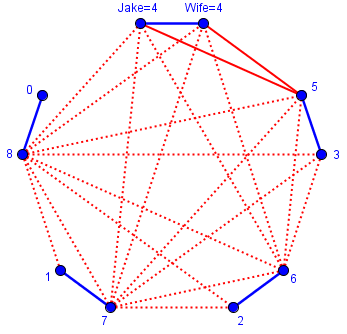Interestingly, each couple shook a total of 8 hands. I don’t know if there is a quick way to have seen this.

## How many men and women?

The next problem is really just algebra, but interesting to consider along with the others. It comes from 1996:

Shaking Hands - How many were at the party?

A group of people met at a party. Each person shook hands with everyone else. Mr. Li shook hands with 3 times as many men as women. Mrs. Li shook hands with 4 times as many men as women. How many men and women were there at the party?

Great problem. The thing to keep in mind is that Mr. Li does not shake hands with himself, nor Mrs. Li with herself.  Therefore the number of men should be equal to 3 times the number of women PLUS 1.  The number of women therefore must be ONE MORE THAN 1/4 the number of men.  Let's set w = no. of Women and m = no. of Men.  Then we can set up two equations:

m = 3w+1  and   w = (m/4)+1

Here we’re assuming that the Li’s did shake hands with each other, taking what it says literally. (Otherwise, they would have shaken hands with exactly the same people, and what is described could not be true.)

The first equation could have been written as $$m-1 = 3w$$, meaning that the number of men, minus Mr. Li, is three times the number of women; and the second equation could be $$m = 4(w-1)$$, since the number of men is four times the number of women, excluding Mrs. Li.

From the first equation, we can solve for w to get:
w = (m - 1)/3
and substitute this into our second equation to get:
(m - 1)/3 = (m/4) + 1

Let's simplify the right side by changing the 1 to 4/4 and adding. Now we have:
(m - 1)/3 = (m + 4)/4

(you'll want to work out the steps if this is not obvious).

Now use the cross-products to obtain:
4m - 4 = 3m + 12

Solve for m and you'll see that there are 16 men.  Substitute back into the second equation and you'll find the number of women.

Using my versions of the equations (to avoid fractions), we might have chosen instead to eliminate m first, replacing m in the first equation with $$m = 4(w-1)$$ to obtain $$4(w-1)-1=3w$$. This simplifies to $$4w-5=3w$$, so that $$w=5$$, and $$m = 4(w-1) = 4(5-1) = 16$$.

Therefore there are 16 men and 5 women (including the Li’s), for a total of 21 people at the party. Here I only have to show Mr. and Mrs. Li’s handshakes, with men in blue and women in red: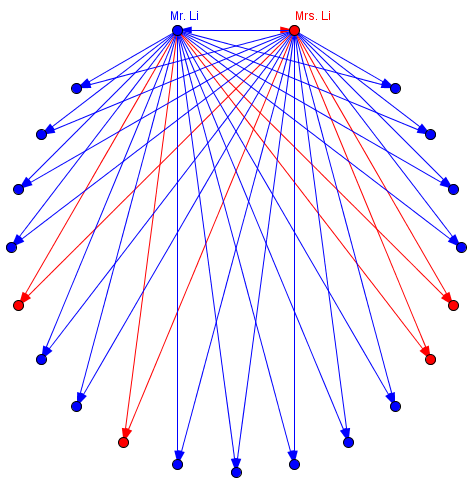Mr. Li shook hands with 15 men and 5 women (a 3:1 ratio), while Mrs. Li shook hands with 16 men and 4 women (a 4:1 ratio). It worked.

You might also want to use this information to determine the TOTAL number of handshakes. You can find it by examining Pascal's Triangle.

I had fun with this one!   Thanks!

The mention of Pascal’s Triangle refers to the fact that it contains binomial coefficients, which count combinations; in particular, we need $${{21}\choose{2}} = \frac{21\cdot 20}{2\cdot 1} = 210$$ handshakes in all. If you like, you might calculate the number of male-male, female-female, and male-female shakes! (Careful; there’s a little twist.)

## Even and odd handshakes

Now we have a proof problem, from 2001, which again is reminiscent of the “Handshake Problem” but is entirely different. In fact, it is what we call a “graph theory” problem, dealing with dots (nodes) joined by lines (edges)

Odd Number of Hands, Even Number of People

Every person on earth has shaken a certain number of hands. Prove that the number of persons who have shaken an odd number of hands is even.

I have played with n and n-1 from the more classic handshake problems, but they do not seem to apply.  I am now starting down the road that for every 1 handshake there are two people involved. Is it that easy, that for any number of handshakes a multiple of two people is involved?

It can be almost that easy, but it needs clear reasoning to become a proof. We’ll get to that, but not just yet!

Before we start, here is a random example representing a group of people who have shaken hands, though not with everyone: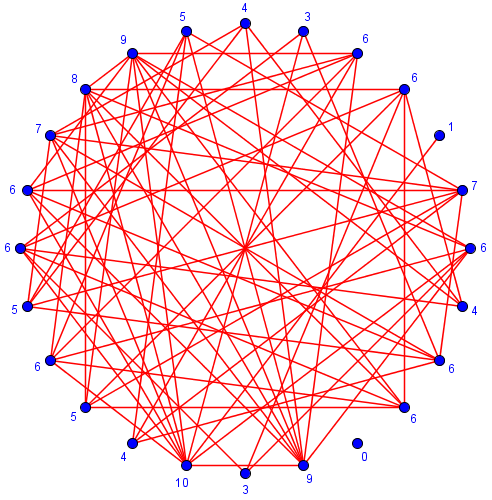If you count (and, yes, I did), you will find that there are 66 handshakes (edges) among 24 people (nodes), 14 have shaken hands with an even number of people (called even nodes), while 10 have shaken hands with an odd number (odd nodes). As claimed, the number of odd nodes is even. What I have done is to make a “graph” representing the problem.

Hi Telinda,

o.....o

In fact, the number of people who have shaken an odd number of hands is even. So far, so good.  Now add a handshake:

o.....o
.
.
o

As before, two people have shaken an odd number of hands. From now on, we have two ways to add a handshake: (1) add another person, or (2) add a handshake between two people who haven't yet shaken hands.

What we’re doing here is, in part, just “playing” with the problem using small numbers, to see how things work; while also starting in the direction of a possible inductive proof, building up to any number of shakes one by one. Or, rather, we are proving that the parity (oddness or evenness) of the number of odd shakes is an invariant, unaffected by any step you take in building up a set.

Also, for brevity, let's let K stand for the number of people who have shaken an odd number of hands.

In case (1), the new person has shaken one hand, so K increases by 1. What about the person he shakes hands with? If that person had previously shaken an even number of hands, now he's shaken an odd number, so K increases by 1 again. So if K was even before, it's even again:

K is even -> K + 2 is even

If that person had previously shaken an odd number of hands, now he's shaken an even number of hands, so K decreases by 1.  So if K was even before, it's even again:

K is even -> K + 1 - 1 is even

I'll let you chase down the details of case (2).

Here is my example above with one node (and handshake) added on the left, and another on the right, showing the two cases: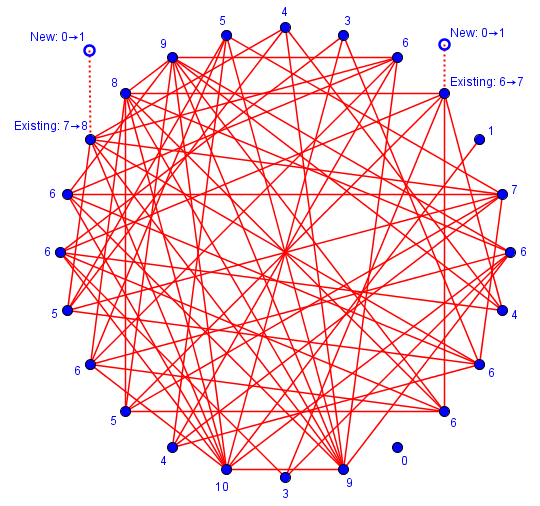The one on the left changed an odd node to even, but added a new odd node, leaving K unchanged; the one on the right changed an even node to odd while adding a new odd node as well, increasing K by 2 and keeping it even.

How about when we add a new edge (handshake) between two existing nodes? If they are both even, both change to odd, changing $$K$$ to $$K + 2$$ which is still even; if they are both odd, both change to even, changing $$K$$ to $$K – 2$$; and if one is odd and the other even, they change places, leaving $$K$$ unchanged. In any case, it is still even.

Here are examples of all three cases: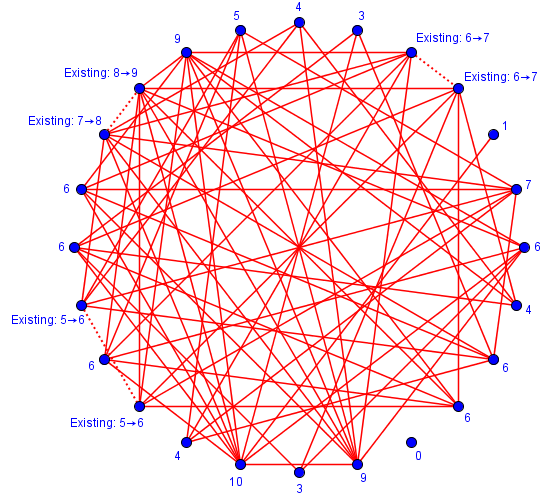## A quicker proof

Even - Odd Handshake Problem

Dr. Math,

Every person on the earth has shaken a certain number of hands. Prove that the number of persons who have shaken an odd number of hands is even.

I understand that this problem is true from experimental research I conducted on paper. However, I am unsure how to prove this problem works for every situation. Could you please help me to prove it? I would really appreciate it.

Thanks!

Experimentation is a good idea; but a proof is needed. Doctor Wilkinson answered:

Very cute problem, Josh!

If you add up the number of hands each person has shaken, you have to end up with an even number, because every handshake gets counted twice. Now the sum of any number of even numbers is even, and the sum of an odd number of odd numbers is odd, and the sum of an even number and an odd number is odd; so the number of persons who have shaken an odd number of hands must be even, not odd.

This appears to be Telinda’s idea from the last question! Since the total number of “hands” is even, there can’t be an odd number of shakes.

And this brings us full circle, as in the counting problems last week, “every handshake gets counted twice” was a key factor.

This site uses Akismet to reduce spam. Learn how your comment data is processed.